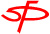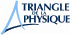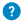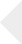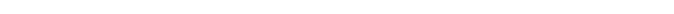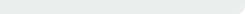Josephson effect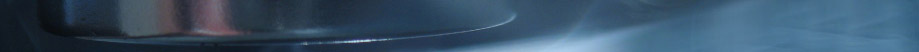# Josephson effect

## Ignoring the frontiers

When two superconductors are separated by a very thin insulating layer, quite unexpectedly, a continuous electric current appears, the value of which is linked to he characteristics of the superconductors. This effect was predicted in 1962 by Brian Josephson. Since then, this superconductor-insulator-superconductor sandwich has been called a “Josephson junction”. Where does such an effect come from?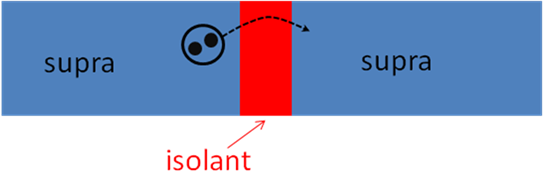When a material becomes superconducting, the electrons form Cooper pairs and condensate in the shape of a unique collective quantum wave. If the electric insulator separating the two superconductors is very thin (only a few nanometres), then the wave can somehow spill out of the superconductor, which enables the electron pairs to go through the insulator thanks to a quantum effect called tunneling effect. When spontaneously going from one superconductor to the other, the pairs create an electric current. Each superconductor is characterized by a quantity called phase, with a subtle signification. The electric current in the junction is a continuous current, the value of which is proportional to the sine of the phase difference between the two superconductors.

Now, if we apply a constant electric tension difference between the two superconductors, an alternating electric current appears, in reaction to the phase variations. This effect that links a continuous voltage to an alternating current is unusual. Especially since the frequency of alternating currents depends neither on the size of the superconductors, nor on their properties (critical temperature, chemical composition). This frequency only depends on the applied voltage and on fundamental permanent features (the electric charge of the electron and Planck quantum of energy). We can measure a frequency very precisely thanks to atomic clocks, but until this effect was discovered, we could not precisely measure a voltage. The Josephson effect enables us to define a reference value of the voltage that is then used to calibrate the measuring devices and to make sure that one volt has the same value in France and in Japan.

Josephson effects are very sensitive to the value of the magnetic field, because the phase variation of a superconductor can be linked to the magnetic flux. It then becomes possible to use this magnetic field sensitivity to build very accurate magnetic field measuring devices, called squids: these devices are the most precise means to measure a magnetic field.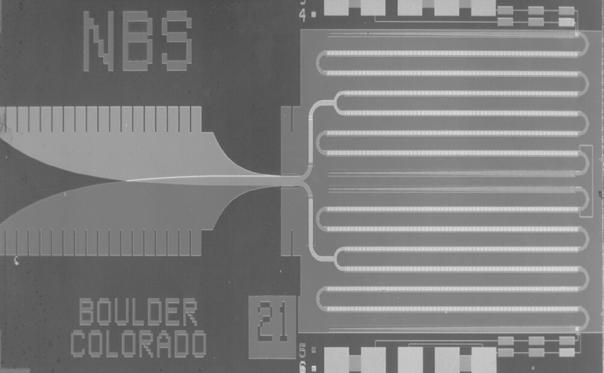This circuit, containing 3020 Josephson junctions, enables to very precisely generate a reference voltage of 1 volt, and can be used to calibrate measuring devices.
National Institute of Standards and Technology, USA